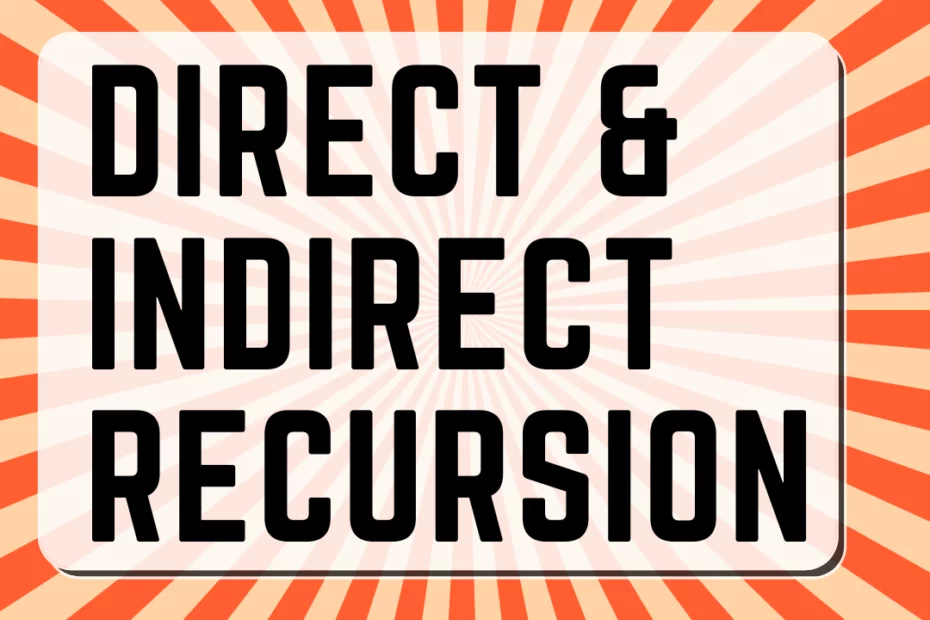# Direct & Indirect Recursion

• DSA

In programming, Recursion means a function calling itself. The best use of recursion is when we have a big/complex problem and is solved by breaking down the problem into smaller instances. In this post, we’ll see direct and indirect recursion.

## Types of Recursion

Generally, there are two main types of Recursion based on whether the function calls itself or calls another,

• Direct Recursion
• Indirect Recursion

## Direct Recursion

As in the name itself, direct recursion is a single step recursion case where the function calls itself.

Example

``````#include <stdio.h>
void fun(int n)
{
if (n > 0)
{
printf("% d", n);
fun(n - 1);
}
}

int main()
{
int x = 5;
fun(x);
return 0;
}``````

Output

``5 4 3 2 1``

## Indirect Recursion

In simple words, indirect recursion depends on another function. It contains two functions that depend on one another.

Example

``````#include<stdio.h>

void funA(int n)
{
if(n>0)
{
printf(" %d",n);
funB(n-1);
}
}

void funB(int n)
{
if(n>0)
{
printf("%d",n);
funA(n-1);
}
}

int main()
{
funA(10);
return 0;
}
``````

Output

`` 10 9 8 7 6 5 4 3 2 1``

## Conclusion

This post is a part of my #30DaysChallenge to write a blog post every day on what I learn. All the Best ~ Abhiram Reddy